Anúncio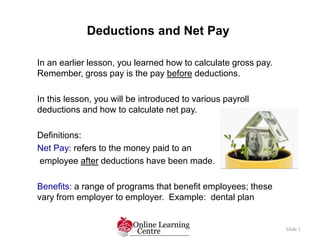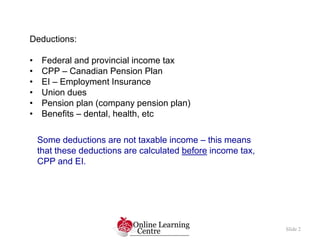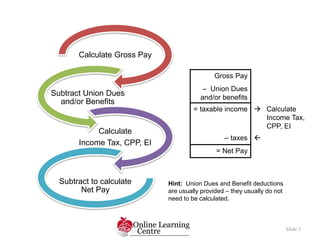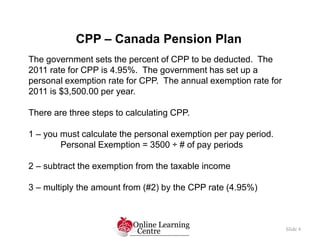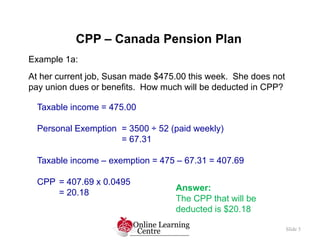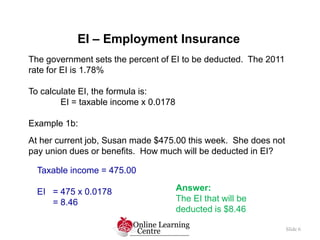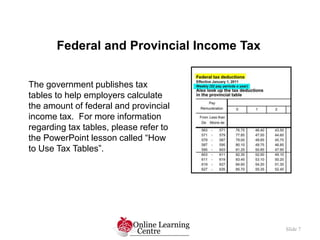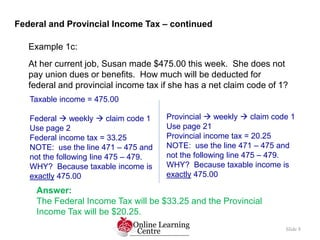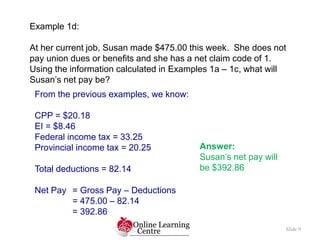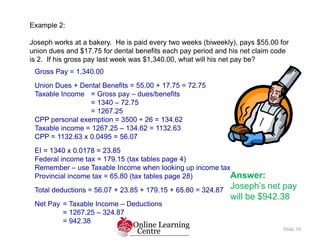1 de 10
Anúncio

### MWA10 2.4 Deductions

1. Deductions and Net Pay Slide 1 In an earlier lesson, you learned how to calculate gross pay. Remember, gross pay is the pay before deductions. In this lesson, you will be introduced to various payroll deductions and how to calculate net pay. Definitions: Net Pay: refers to the money paid to an employee after deductions have been made. Benefits: a range of programs that benefit employees; these vary from employer to employer. Example: dental plan
2. Slide 2 Deductions: • Federal and provincial income tax • CPP – Canadian Pension Plan • EI – Employment Insurance • Union dues • Pension plan (company pension plan) • Benefits – dental, health, etc Some deductions are not taxable income – this means that these deductions are calculated before income tax, CPP and EI.
3. Slide 3 Calculate Gross Pay Subtract Union Dues and/or Benefits Calculate Income Tax, CPP, EI Subtract to calculate Net Pay Gross Pay – Union Dues and/or benefits = taxable income  Calculate Income Tax, CPP, EI – taxes  = Net Pay Hint: Union Dues and Benefit deductions are usually provided – they usually do not need to be calculated.
4. Slide 4 The government sets the percent of CPP to be deducted. The 2011 rate for CPP is 4.95%. The government has set up a personal exemption rate for CPP. The annual exemption rate for 2011 is \$3,500.00 per year. There are three steps to calculating CPP. 1 – you must calculate the personal exemption per pay period. Personal Exemption = 3500 ÷ # of pay periods 2 – subtract the exemption from the taxable income 3 – multiply the amount from (#2) by the CPP rate (4.95%) CPP – Canada Pension Plan
5. Slide 5 Example 1a: At her current job, Susan made \$475.00 this week. She does not pay union dues or benefits. How much will be deducted in CPP? CPP – Canada Pension Plan Taxable income = 475.00 Personal Exemption = 3500 ÷ 52 (paid weekly) = 67.31 Taxable income – exemption = 475 – 67.31 = 407.69 CPP = 407.69 x 0.0495 = 20.18 Answer: The CPP that will be deducted is \$20.18
6. Slide 6 The government sets the percent of EI to be deducted. The 2011 rate for EI is 1.78% To calculate EI, the formula is: EI = taxable income x 0.0178 Example 1b: At her current job, Susan made \$475.00 this week. She does not pay union dues or benefits. How much will be deducted in EI? EI – Employment Insurance Taxable income = 475.00 EI = 475 x 0.0178 = 8.46 Answer: The EI that will be deducted is \$8.46
7. Slide 7 The government publishes tax tables to help employers calculate the amount of federal and provincial income tax. For more information regarding tax tables, please refer to the PowerPoint lesson called “How to Use Tax Tables”. Federal and Provincial Income Tax
8. Slide 8 Example 1c: At her current job, Susan made \$475.00 this week. She does not pay union dues or benefits. How much will be deducted for federal and provincial income tax if she has a net claim code of 1? Federal and Provincial Income Tax – continued Answer: The Federal Income Tax will be \$33.25 and the Provincial Income Tax will be \$20.25. Taxable income = 475.00 Federal  weekly  claim code 1 Use page 2 Federal income tax = 33.25 NOTE: use the line 471 – 475 and not the following line 475 – 479. WHY? Because taxable income is exactly 475.00 Provincial  weekly  claim code 1 Use page 21 Provincial income tax = 20.25 NOTE: use the line 471 – 475 and not the following line 475 – 479. WHY? Because taxable income is exactly 475.00
9. Slide 9 Example 1d: At her current job, Susan made \$475.00 this week. She does not pay union dues or benefits and she has a net claim code of 1. Using the information calculated in Examples 1a – 1c, what will Susan’s net pay be? From the previous examples, we know: CPP = \$20.18 EI = \$8.46 Federal income tax = 33.25 Provincial income tax = 20.25 Total deductions = 82.14 Net Pay = Gross Pay – Deductions = 475.00 – 82.14 = 392.86 Answer: Susan’s net pay will be \$392.86
10. Slide 10 Example 2: Joseph works at a bakery. He is paid every two weeks (biweekly), pays \$55.00 for union dues and \$17.75 for dental benefits each pay period and his net claim code is 2. If his gross pay last week was \$1,340.00, what will his net pay be? Gross Pay = 1,340.00 Union Dues + Dental Benefits = 55.00 + 17.75 = 72.75 Taxable Income = Gross pay – dues/benefits = 1340 – 72.75 = 1267.25 CPP personal exemption = 3500 ÷ 26 = 134.62 Taxable income = 1267.25 – 134.62 = 1132.63 CPP = 1132.63 x 0.0495 = 56.07 EI = 1340 x 0.0178 = 23.85 Federal income tax = 179.15 (tax tables page 4) Remember – use Taxable Income when looking up income tax Provincial income tax = 65.80 (tax tables page 28) Total deductions = 56.07 + 23.85 + 179.15 + 65.80 = 324.87 Net Pay = Taxable Income – Deductions = 1267.25 – 324.87 = 942.38 Answer: Joseph’s net pay will be \$942.38
Anúncio